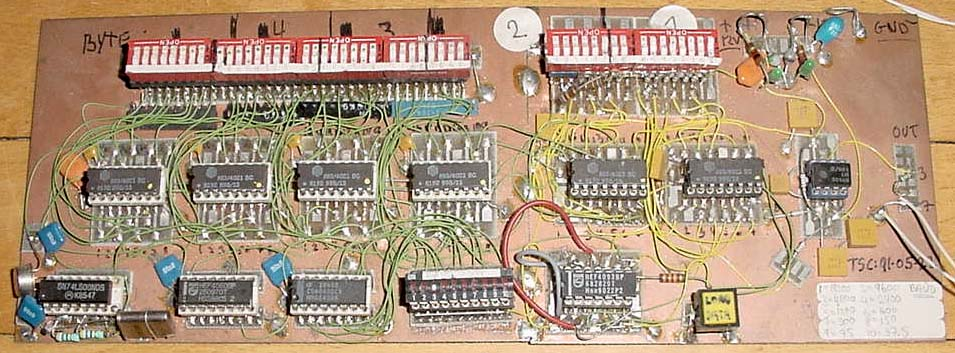www.webx.dk Start pageOZ2CPU Radioamateur mainpageHomemade electronics Stuff old and newSerial RS-232 senderThe 6 pcs 4021 is parallel to serial converters, they are cascaded so that a 48bits signal can be made
The clock is made using a 2.4576 Mhz crystal and a 74LS00 for the osc.
This clock is divided first in a 4060 and then in a 4040,
via the 10 position dil switch clock signals can be selected:
1=19200 2=9600 3=4800 4=2400 5=1200 6=600 7=300 8=150 9=75 10=37.5 BAUD

The 5 volt output from the last 4021 is converted into RS-232 levels via an LM-301 opamp
This construction was made 22 may 1991 and it still works :-)